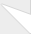# C++ Operators

First of all, what is an operator? There are multiple types of operators, but in this tutorial we'll only talk about 2 types. The first type includes arithmetic operators such as addition, subtraction, multiplication, and division. The second type includes equals, greater than, and other comparison testing operators.

First, lets talk about arithmetic operators, simply put the operator between two variables you want to operate on:

+ is addition
- is subtraction
* is multiplication
/ is division
++ increase number by 1
-- decrease number by 1

These operators can be used in different ways. Sometimes other data types such as string can use the addition operation, because it overloads it as a "concatenation" (combining) operation (you cannot do addition with strings). Here's an example of using all the operators:
#include <iostream>
#include <string>

using namespace std;

int main(){
int number = 5 + 5 / (3 * 2) - 2; // a math equation
number = number % 2; // modulus, gets the remainder of number divided by 2.
number %= 2; // Same operation as above.
string thee("The");
string tee("Fox");
number++; // save and then increment by 1.
++number; // increment by 1 and save.
thee += " " + tee;
thee += " ran incredibly fast through the forest.";
cout << thee << endl; // "Fox ran incredibly fast through the forest.";
return 0;
}

You will most likely only ever use these in if, while, do, and for statements, or in loops. The operators look like this:

== is equal to
!= is not equal to (equivalent to <>)
> is greater than
< is less than
>= is greater than or equal to
<= is less than or equal to

Here is an example of using comparison operators in an if statement.

if(((5 + 5) / 2) >= 3){
cout << "10/2 = 5, and five IS greater than or equal to ten, so this text will display.";
}### Thanks for this, this helpedThanks for this, this helped me with homework very much